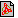Cím: Problems of the 1986 Kürschák József Competition Füzet: 1987/február, 80. oldalPDF file Témakör(ök): Kürschák József (korábban Eötvös Loránd)

A szöveg csak Firefox böngészőben jelenik meg helyesen. Használja a fenti PDF file-ra mutató link-et a letöltésre.

Problems of the 1986 Kürschák József Competition
1. Prove that three semi lines starting from a given point contain three face diagonals of a cuboid if and only if the semi lines include pairwise acute angles such that their sum is ${180}^{\circ }$.
2. Let us assume that $n$ is a positive integral number greater than two. Find the maximum value for $h$ and the minimum value for $H$ such that

 $\begin{array}{c}h<\frac{{a}_{1}}{{a}_{1}+{a}_{2}}+\frac{{a}_{2}}{{a}_{2}+{a}_{3}}+\text{...}+\frac{{a}_{n}}{{a}_{n}+{a}_{1}}
holds for any positive numbers ${a}_{1}$, ${a}_{2}$, $\text{...}$, ${a}_{n}$.
3. $A$ and $B$ play the following game. They arbitrarily select from among the first $100$ positive integral numbers $k$ ones. If the sum of the selected numbers is even then $A$ wins, if their sum is odd then $B$ is the winner. For what values of $k$ are equal the chances for $A$ and $B$?